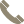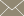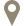Home » Faculty Profiles » Faculty

### Saikali, Norma

Instructional Assistant Professor

#### CONTACT INFO(956) 326-2598norma.saikali@tamiu.eduLBV-313

#### COURSES TAUGHT

• Advanced Topics in Mathematics (MATH 5355)
• Anlys of Var in Expt Dsgn Mdls (STAT 5329)
• Business Math I (MATH 1324)
• Calculus I (MATH 2413)
• Calculus II (MATH 2414)
• Calculus III (MATH 2415)
• College Algebra (MATH 1314)
• Fundamentals of Mathematics II (MATH 1351)
• Geometry (MATH 3325)
• Geometry of Curves&Surfaces (MATH 4365)
• Introduction to Linear Algebra (MATH 3310)
• Introductory Statistics (MATH 1342)
• Pre-Calculus (MATH 2412)
• Probability (MATH 5375)
• Real Analysis I (MATH 5305)
• Selected Topics in Math (MATH 4355)
• Statistical Analysis (MATH 3360)
• Topology (MATH 5365)